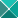Portal OutlookWebApp VPN.kfupm GmailFaisal Fairag, Associate Professor , KFUPM Office: (+9663) 860-4463 KFUPM BOX 1533 Math. Secretary: (+9663) 860-2193 FAX: (+9663) 860-2340 FAX: (+9663) 860-6302 Dhahran,31261 Email: Saudi Arabia Home: (+9663) 860-6302

 Seminars

# Interesting Mathematical Links

###Brief CV:

Ph.D. (1998 University of Pittsburgh), MA (1996 University of Pittsburgh)

MS. (1989 KFUPM), BS (1987 KFUPM)

Associate Professor, 2010-present, Math Dept, King Fahd University of Petroleum & Minerals

Assistant Professor, 1998-2010, Math Dept, King Fahd University of Petroleum & Minerals

Prep. Year Math Program Coordinator, 2001-2003, Prep. Year Math Program, King Fahd University of Petroleum & Minerals

Lecturer, 1989-1998, Math Dept, King Fahd University of Petroleum & Minerals

Graduate Assistant, 1987-1998, Math Dept, King Fahd University of Petroleum & Minerals

###Research:

Faisal Fairag's research interests lie primarily in the field of Numerical Analysis and computational mathematics. He studies numerical methods for partial differential equations and in particular finite element methods. His current major research interest is in Finite Element Methods for the Navier-Stokes equations and Ladyzhenskaya model for incompressible viscous flow. He studies the theoretical analysis, algorithmic developments and practical applications of these methods. Research publications  of Fairag can be fond here.

###Courses

MATH 102 - Calculus II  (term 182)   (Current Term)

MATH 101 - Calculus I  (term 181)

MATH 321 - Introduction to Numerical Computing (term 171)

MATH 101 - Calculus II  (term 162)

MATH 101 - Calculus I  (term 161)

MATH 102 - Calculus II  (term 152)

MATH 571 - Numerical Analysis of Ordinary Differential Equations (term 151)

MATH 101 - Calculus I  (term 151)

MATH 301 - Methods of Applied Mathematics (term 143)

MATH 102 - Calculus II  (term 142)

MATH 102 - Calculus II  (term 141)

MATH 102 - Calculus II  (term 132)

MATH 101 - Calculus I  (term 131)

MATH 102 - Calculus II  (term 122)

MATH 102 - Calculus II  (term 121)

MATH 102 - Calculus II  (term 112)

MATH 101 - Calculus I  (term 111)

MATH 590 - Special Topics in Numerical Linear Algebra  (term 111)

MATH 260 - Introduction to Differential Equations and Linear Algebra  (term 092)

MATH 470 - Partial Differential Equations   (term 091)

MATH 260 - Introduction to  Differential Equations and Linear Algebra  (term 082)

MATH 572 - Numerical Analysis of Partial Differential Equations  (term 081)

MATH 301 - Methods of Applied Mathematics (term 061)

MATH 202 - Elements of Differential Equations (term 052)

MATH 101 - Calculus I (term 031)

MATH 574 - Numerical Methods for Partial Differential Equations (Term-022)

MATH 101 - Calculus I  (term 011)

MATH 102 - Calculus II

MATH 260 - Linear Algebra and Differential Equations

MATH 574 - Numerical Methods for Partial Differential Equations

MATH 321 - Numerical Mathematics & Computing

MATH 280 – Linear Algebra

MATH 202 - Elements of Differential Equations

###Useful

?          SIAM

?          American Mathematical Society

?          The Mathematical Association of America

?          European Mathematical Information Service

?          Mathematics Subject Classification 2000

?          Classical Analysis (CA) section of the xxx math archives.

?          Zentralblatt MATH Database

?

?          Some Math Links by Prof. William Layton

?         Scopus (through kfupm library: search for Scopus )

?         KFUPM Library  ( InterLibraryLoan Request)

?         EBSCO Discovery Service (EDS)

?         MathSciNet

?         Deanship of Scientific Research

####Journals

?          ETNA Electronic Transactions on Numerical Analysis

?          Journal of Symbolic Computation

?          SIAM journals

?          Electronic Research Announcements of the AMS

?          IDEAL

####Publishers

?          Springer-Verlag

?          Cambridge University Press

?          Oxford University Press

?          Elsevier

?          World Scientific

?          Institute of Physics

?          Birkhauser

####Software

?          MATLAB

?          Available Mathematical Software

?          TeX archives

?          Maple

?          Wolfram (Mathematica)

?          Personal TeX

####Others

?          Fortran Library

?          IFER - Internet Finite Element Resources

?           YES 2000, Yosemite Educational Symposium, October 29 - November 1, 2000, Yosemite National Park

?         الدورة الاولى لمدرسي الرياضيات

?          Gmail

?

?         SCOPUS via KFUPM

modified by Faisal Fairag,